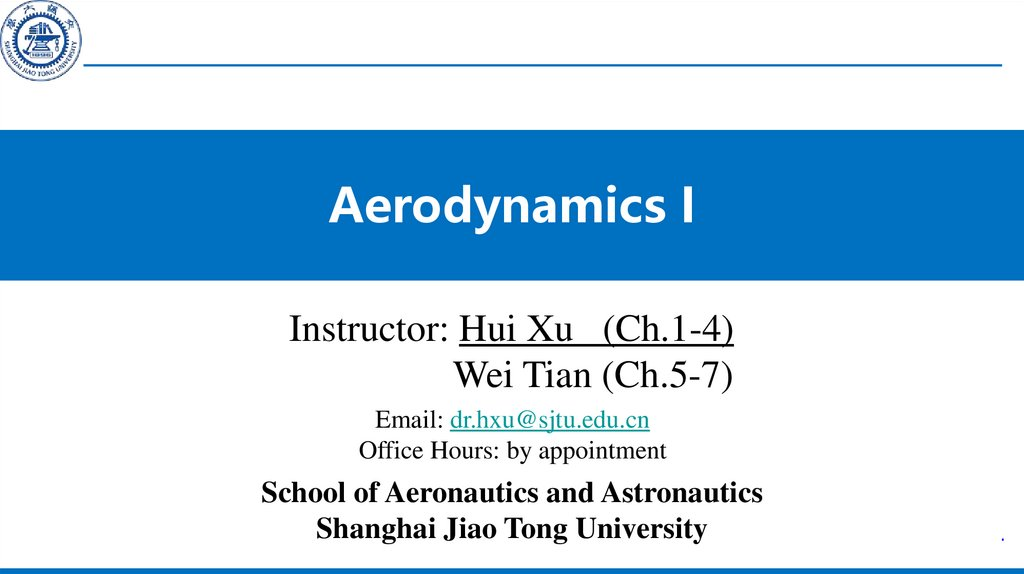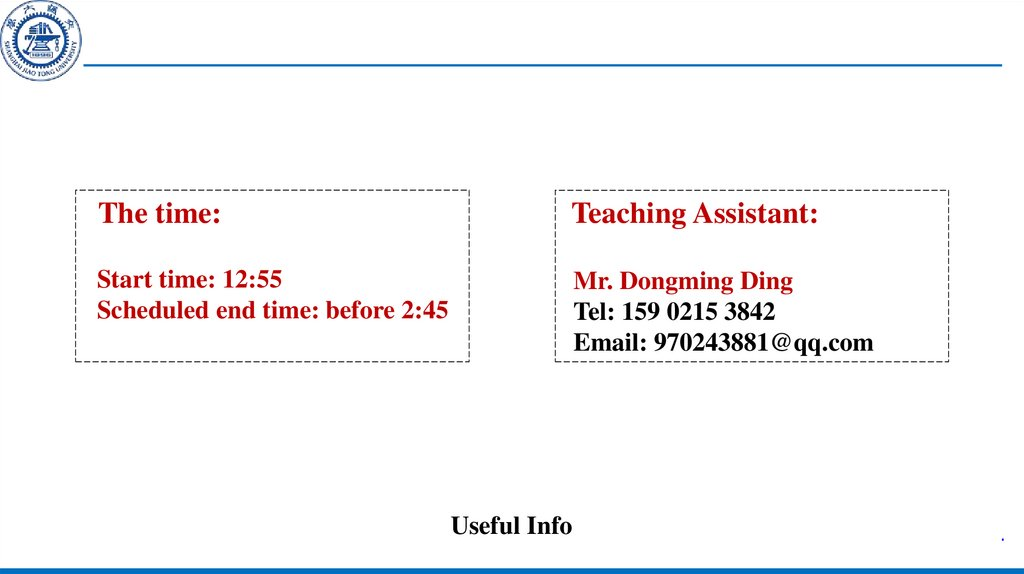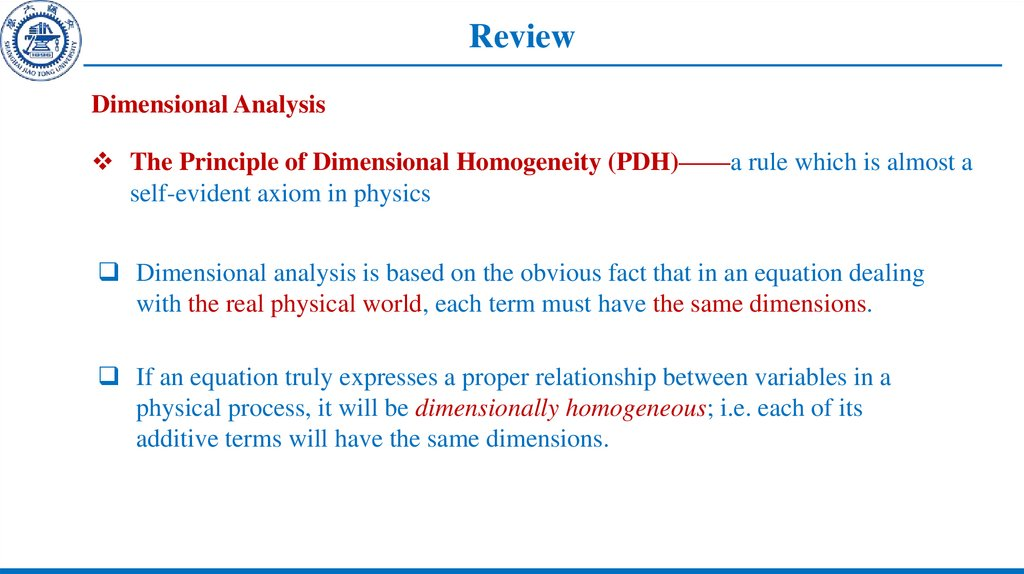# Aerodynamics I

## 1.

Aerodynamics I
Instructor: Hui Xu (Ch.1-4)
Wei Tian (Ch.5-7)
Email: [email protected]
Office Hours: by appointment
School of Aeronautics and Astronautics
Shanghai Jiao Tong University

## 2.

The time:
Teaching Assistant:
Start time: 12:55
Scheduled end time: before 2:45
Mr. Dongming Ding
Tel: 159 0215 3842
Email: [email protected]
Useful Info

## 3. Review

Dimensional Analysis
The Principle of Dimensional Homogeneity (PDH)——a rule which is almost a
self-evident axiom in physics
Dimensional analysis is based on the obvious fact that in an equation dealing
with the real physical world, each term must have the same dimensions.
If an equation truly expresses a proper relationship between variables in a
physical process, it will be dimensionally homogeneous; i.e. each of its
additive terms will have the same dimensions.

Basic Theorem

## 5. Review

Flow similarity
By definition, different flows are dynamically similar if:
1. The streamline patterns are geometrically similar.
2. The distributions of V/V∞, p/p∞, T/T∞, etc., throughout the flow field are
the same when plotted against common non-dimensional coordinates.
3. The force coefficients are the same.

## 6. Chapter 1: Introduction

Wind-tunnel test
Schematic of the variable density tunnel (VDT) (From Baals, D. D. and Carliss, W.
R., Wind Tunnels of NASA, NASA SP-440, 1981).

## 7. Review

Fluid static dynamics: Buoyance
Consider the forces along y axis: Positive upward, then—
Hydrostatic equation — differential equation, which connects the pressure variation in fluid with the vertical
height.

## 8. Review

Type of flow
• Continuum flow
If the orders of magnitude of λ is smaller than the specific scale of body(such as D)
relatively, and then the fluid behaves as a continuous medium. The fluid presents a
macro-behaving property, why?
• Free molecular flow
If λ is the same order of the specific scale of body the fluid presents individual
molecules’ behaving.
• Intermediate state
The flow features are between the above two — “Low density flow”
• Most practical aerodynamic applications involve continuum flow.

## 9. Review

Inviscid flow versus Viscous flow
Viscous flow: This transport on a molecular scale gives rise to the phenomena of mass diffusion,
viscosity (friction), and thermal conduction. All real flows exhibit the effects of these transport
phenomena, such flows are called viscous flows.
Inviscid flow: Really exists? Inviscid flows do not truly exist in nature.

## 10. Course Diagram

Fundamental of Aerodynamics
Introduction
Fundamental principles and equations
Inviscid, incompressible flow
Inviscid,
compressible flow
Viscous flow
Fundamental principles and
equations
Preliminaries
Fundamental principles and
equations
Shock
Boundary layer
Two-dimensional flows
Flow over finite wing
Quasi-onedimensional flow
Three dimensional flows
Two-dimensional
flow

Linearized, nonlinear flow
Hypersonic flow

## 11. Chapter 1

Learning Targets
• Viscous Flow: Boundary layers
• Applied Aerodynamics: The Aerodynamic Coefficients
• Integrated Work Challenge: Forward-facing axial force

## 12. Chapter 1: Introduction

1.11 Viscous flow: Brief introduction on
boundary layer
• At any point in flow field, provided that
the velocity gradient exists, the shear
stress does also.
• Only where the gradients are substantial,
has the local stress as a meaningful effect
on the flow.
• The flow field can be divided into two
regimes—
1. Inside a thin layer adjacent to the body surface, the velocity gradient is large,
friction play a definitive/crucial role—Viscous flow (Boundary layer)
2. In the region outside the thin layer, the gradient is so relatively small that
the friction plays nearly no role—Inviscid flow

## 13. Chapter 1: Introduction

• In 1904, Prandtl put forward the concept of
boundary layer: boundary layer—the region
adjacent to the body surface. Two regions—
viscous and inviscid, can be solved respectively.
• Flow separation — Pressure distribution will be
changed severely—thin, but important
Pressure drag

## 14. Chapter 1: Introduction

• Question: Why are the gradients so large inside the
layer?
• Inviscid, no friction, the surface is streamline. In fact,
adjacent to the surface, the molecules’ velocities are
zero—no-slip condition, but the increasing gradient
must be?
• The boundary layer theory: shear stress τw and layer
thickness δ.
• Indicated by researches: The pressure is nearly
constant along the normal direction to the surface in
the layer.
• Question: What does the above suggest?
The pressure at the outside edge obtained by inviscid theory has no any
change across the layer (the change can be neglected);
• The distribution is very close to the real one(very high accuracy);
• The above conclusions have preconditions: thin layer and no separation.

## 15. Chapter 1: Introduction

Note: the above two are reasonable for the layer attached on the surface all the way, but
not appropriate for the separated flow shown by Fig.1.43.
• Velocity profile inside boundary layer: The curve (vector end curve) of the velocity
inside the boundary layer as the function of the normal axis to the local body surface.
Function of x.

## 16. Chapter 1: Introduction

Temperature profile through the layer: The
curve of the temperature through the
boundary layer as the function of the local
normal axis. Dominated by (1) Thermal
conduction——heat mixing by molecules
random motion; (2) Frictional dissipation—
—frictional shear stress results in energy
conversion(kinetic- internal).
The shear stress on the wall skin is
determined by the slope rate of the velocity
where, μ is the absolute viscous coefficient
(M/LT).

## 17. Chapter 1: Introduction

• μ variation with temperature: liquid (olive ;
soybean- edible oil)—T increase, μ decrease;
Gas, the contrary. Sea level, standard
atmospheric air (right figure: μ= μ(T))
μ=1.7894×10-5kg/(m)(s)
• The slope of the temperature profile on the
wall indicates whether if the wall is aeroheated or cooled. Aero-heating rate is:
Where, k is the thermal conductivity of the
gas. The negative sign ?

## 18. Chapter 1: Introduction

• At standard sea level temperature:
k=2.53×10-2J/(m)(s)(K)
• Proportional to the viscous coefficient essentially, i.e
k=(const) ×μ
• “Convective heat transfer”: the air flow over a body surface heats or
cools the surface(thermal conduction).
• “Aerodynamic heating”: the heat transfer between boundary layer and
body surface. Dominates the supersonic flow especially hypersonic one.

## 19. Chapter 1: Introduction

• Re effect on boundary layer:
• Figure below: Development of boundary layer on plate
Local Reynolds number at x away from the leading edge(characteristic length is x):
• “∞” dictates the parameter of the far forward flow relative to plate.
• The skin friction(shear stress) and the thickness of boundary layer at x on the plate
are the function of Rex .

## 20. Chapter 1: Introduction

• The Reynolds number generally
governs the nature of the viscous
flow.
• The basic types of viscous flow:
1. Laminar flow: the streamlines
are smooth and regular , and the
fluid element moves smoothly
along the streamline;
2. Turbulent flow: the streamlines
break up and a fluid element
moves in a random, irregular
and tortuous fashion.

## 21. Chapter 1: Introduction

Fig.1.53: Comparison for the two
velocity profiles——
1. Turbulent, relatively “plump”
Approaching to the wall, the
gradients of the two flows are different.
The relation of the velocity gradient
at the wall:

## 22. Chapter 1: Introduction

• By Eq.1.59: (τw)laminer<(τw)turbulent
The important fact: The shear stress of laminar flow is smaller than turbulent flow !
The types of the wall boundary layer determine the character of the frictional force
on the aircraft——the frictional drag produced by laminar flow is smaller relatively!
There is similar result for “aero-heating”: Turbulent flow is larger than laminar flow,
even much huge. For hypersonic flow, even up to more than 10 times!

## 23. Chapter 1: Introduction

Homework: 1.11, 1.12
Questions for thinking:
1. After released, the hydrogen ball will continue to go up, what will happen in
the end? Please analyze the reason;
2. Deduce the Pascal law by Hydrostatic equation.

## 24. Chapter 1: Introduction

1.12 Applied aerodynamics: Aerodynamic coefficients——
Magnitudes and variations
Applied aerodynamics: For the practical evaluation on the aerodynamic properties of
aircrafts and design works.
(1) Configuration and performance; (2) Properties of flow field; (3) Components
design; (4) Drag reducing design; (5) Type modified design;(6) New conceptual
aircraft , etc.
In fact, aerodynamics evaluations run through the whole developing process of aircraft,
and are gradually coupled with the analysis for every other subjects(such as structural
dynamics or flight dynamics etc.) tightly, in order to solve conceptual design,
components design, aero-elastic design, flight quality( maneuverability, stability),
structural strength( fatigue lifetime), heath monitor, human factor and other problems.

## 25. Chapter 1: Introduction

• Lift, Drag and moment coefficient are the most frequently used technical terms in
outflow aerodynamics.
• It is very important to master the magnitude concept of the actual typical values of
aerodynamic coefficients.
• Question: Is it meaningful for drag coefficient to take the value in [10-5,1000] ?
• The order of magnitude of the aerodynamic coefficients for commonly used
configurations are listed below.
• Question: What are the typical drag coefficients of different aerodynamic
configurations?

## 26. Chapter 1: Introduction

• Fig.1.54, Typical drag coefficients of
some bodies (fixed attitude) moving in a
low speed.
• By Sec. 1.7:
CD =f(Re, M∞) , then, CD =f(Re) .
• Precondition for simplification ?
• Re = ?
• Comparisons between Case a, b and c:
Re all are 105, specific lengths d, and
the specified areas are S=d·1. From a to c,
the wake area shrinks and decreases,
while the drag coefficient becomes
smaller. CD=2——1.2——0.12.

## 27. Chapter 1: Introduction

• Comparisons between Case b and d :
One order of magnitude difference in
both Re and diameter, but CD same, all
are 1.2. For cylinders, Re in the domain
of 104-105, CD values are relatively not
inflected by the Reynolds number.
• Value of drag?

## 28. Chapter 1: Introduction

• Comparisons between Case c and d :
Same dynamic pressures, drags are
same too; c body a streamline one and
height is 10 times of d‘s!
• The drag reducing effect of
streamline one is significant!

## 29. Chapter 1: Introduction

• Comparisons between Case b and e :
• Same diameter, but Ree=100·Reb,
and CDe=2·CDb
——the wake is relatively small !(Part4)

## 30. Chapter 1: Introduction

Note Character parameter(per unit span)—the maximum windward cross-sectional
area. The drag coefficients vary from 2.0 to 0.12. Typical magnitude change.
The Reynolds numbers also change from tens of thousands to tens of millions. At
standard sea level, for circle cylinder,
ρ∞= 1.23kg/m3; µ∞ =1.7894×10-5kg/(m)(s) ; V∞ =45m/s; D=1m
then
In practice, the orders of magnitude of usually used Reynolds number in aerospace are
from millions to tens of millions .

## 31. Chapter 1: Introduction

• Nature of drag: Axial force, Eq.1.8 becomes——
• The aerodynamic drag on any body consists of pressure drag and friction drag .

## 32. Chapter 1: Introduction

Fig.1.55, the comparison for
the relative
quantities of two types of drag of the above
bodies.
Note
1. The drags of upright plate and cylinder are
mainly dominated by pressure drag;
2. The most part of drag of the streamline body
is skin friction drag;
3. ρ∞、μ∞ same, v∞ determined by Re, then v∞
in case e is much larger, and its drag is much
larger than in case b.

## 33. Chapter 1: Introduction

Thus, there are two types of typical aerodynamic shapes:
1. Blunt body: Most part of drag of the body (the radius of its head is
relatively large) are pressure drag;
2. Streamline body: Most part of drag of the body are skin friction drag;
The drag of blunt body is much larger because its flow separates in its
most area.
• Pressure drag: The pressure difference drag resulted by the separated flow,
also called “shape drag”.

## 34. Chapter 1: Introduction

• Variation of the plate drag at zero angle of attach with the Reynolds
number : The drag is totally produced by shear stress, and no any pressure
in drag direction.
• Skin friction drag coefficient:
Where, the reference area is the plane area per unit span.

## 35. Chapter 1: Introduction

The figure listed above indicates that:
1) Cf strongly depends on Re, of which the character length l is the chord length c of
the plate. As Re increases, Cf decreases;
2) Cf value is decided by the flow over the plate whether is laminar or turbulent. With
the same Re, the turbulent is larger than the laminar;
3) Cf The typical magnitude range is from 0.001 to 0.01 in a large Re domain.
The difference relative to upright plate?

## 36. Chapter 1: Introduction

• NACA63-210 airfoil
With thickness, laminar airfoil—low
angle—laminar flow—turbulent one at
higher angle, the drag increases quickly.
Minimum drag exists(0.0045)—“bottom
of pot (barrel)”.
• For typical airfoils — 0.004~0.006
(dominated by friction)
• For the streamline body — flow
separation—pressure drag—the drag
coefficient increases with angle.

## 37. Chapter 1: Introduction

• The drag on a low speed airplane :
Seversky P-35, the typical fighter in
1930s. Fig.1.58 is the detail drag
variation for the aircraft in its design
process.
Note: condition 1 is clean aerodynamic
configuration, CL=0.15, CD=0.0166.
From 2
to 18, conventional and
operational changes to equip the plane ,
all the additions make the drag
coefficient increase up to more than
65%, as all are equipped, it reaches to
0.0275, this is the typical value for this
type of aircraft.

## 38. Chapter 1: Introduction

• Question: As Mach number increases, how
will the drag coefficient change ?
• Northrop T-38A jet trainer, see Fig.1.60.
Zero-lift drag coefficient CDL=0 : The
corresponding drag coefficient when the lift is
just zero for an aircraft at a small angle of
attack.
• Before the quick increase of the drag,
CD≈0.015, lower than that of P35 due to
its good aerodynamic shape. 0.86

## 39. Chapter 1: Introduction

• Typical order of magnitude of lift
coefficient:
Fig.1.61-NACA63-210 airfoil ‘s lift
curve—cl in the range of [-1.0, 1.5],
AOA — -12° to +14°.
• Lift-to-drag ratio L'/D‘(cl / cd ):
Important index; the ratio —
cl / cd =0.6/0.0046=130 4 °
To produce enough lift by
overcoming drag as little as possible!

## 40. Chapter 1: Introduction

• Lift coefficient for full aircraft:
Fig.1.62- CL-α curve for T38 :
added the influence of the flap deflection
angle variation.
• Lift to drag ratio for full aircraft:
About 10. More components and the 3D
flow effect of wing tip—induced drag(extra
pressure drag), thus, much smaller than airfoil.
For B-52 boomer, it has its maximum value
21.6.

## 41. Chapter 1: Introduction

The order of magnitude of moment coefficient :
Fig.1.63-cm.c/4 curve for NACA63-210 airfoil. Mainly negative value, magnitude —
-0.035 or so, typical one.

## 42. Chapter 1: Introduction

Homework: p1.16、p1.18
Thinking work:
Can all the drag come down to pressure drag or friction drag from the view of
body itself ?

## 43. Chapter 1: Introduction

The key points and difficult points in this chapter
Key points:
1. Fundamental aerodynamics variables;
2. Aerodynamic forces and moments
3. Pressure center
4. Similar concepts, theorems, criterion and derived methods
5. Flow type
6. Order of magnitudes of aerodynamic forces
Difficult points:
1. Concept of pressure center
2. Similar criterion and derived methods

Summation

Summation

46